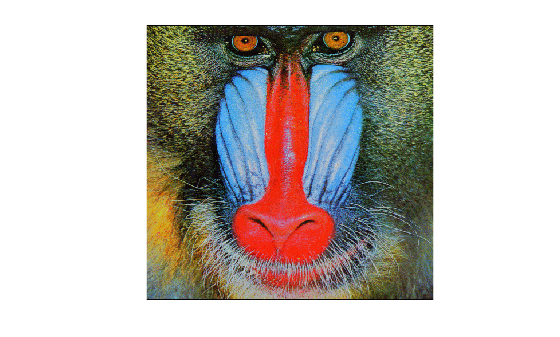# imapprox

## 语法

``[Y,newmap] = imapprox(X,map,Q)``
``[Y,newmap] = imapprox(X,map,tol)``
``Y = imapprox(X,map,inmap)``
``___ = imapprox(___,dithering)``

## 说明

``[Y,newmap] = imapprox(X,map,Q)` 使用具有 `Q` 种量化颜色的最小方差量化法来近似表示索引图像 `X` 和关联颜色图 `map` 中的颜色。`imapprox` 返回索引图像 `Y` 和颜色图 `newmap`。`
``[Y,newmap] = imapprox(X,map,tol)` 使用容差为 `tol` 的均匀量化法来近似表示索引图像 `X` 和关联颜色图 `map` 中的颜色。`
``Y = imapprox(X,map,inmap)` 使用基于颜色图 `inmap` 的逆颜色图映射法来近似表示索引图像 `X` 和关联颜色图 `map` 中的颜色。逆颜色图算法会在 `inmap` 中查找与 `map` 中的颜色最匹配的颜色。`
``___ = imapprox(___,dithering)` 启用或禁用抖动。`

## 示例

```figure load mandrill image(X) colormap(map) axis off axis image``````figure [Y,newmap] = imapprox(X,map,16); image(Y) colormap(newmap) axis off axis image```## 算法

`imapprox` 使用 `rgb2ind` 创建一个使用较少颜色的新颜色图。有关量化法和逆颜色图映射法的详细信息，请参阅 `rgb2ind`算法